Ex 16.1

Chapter 16 Class 8 Playing with Numbers
Serial order wise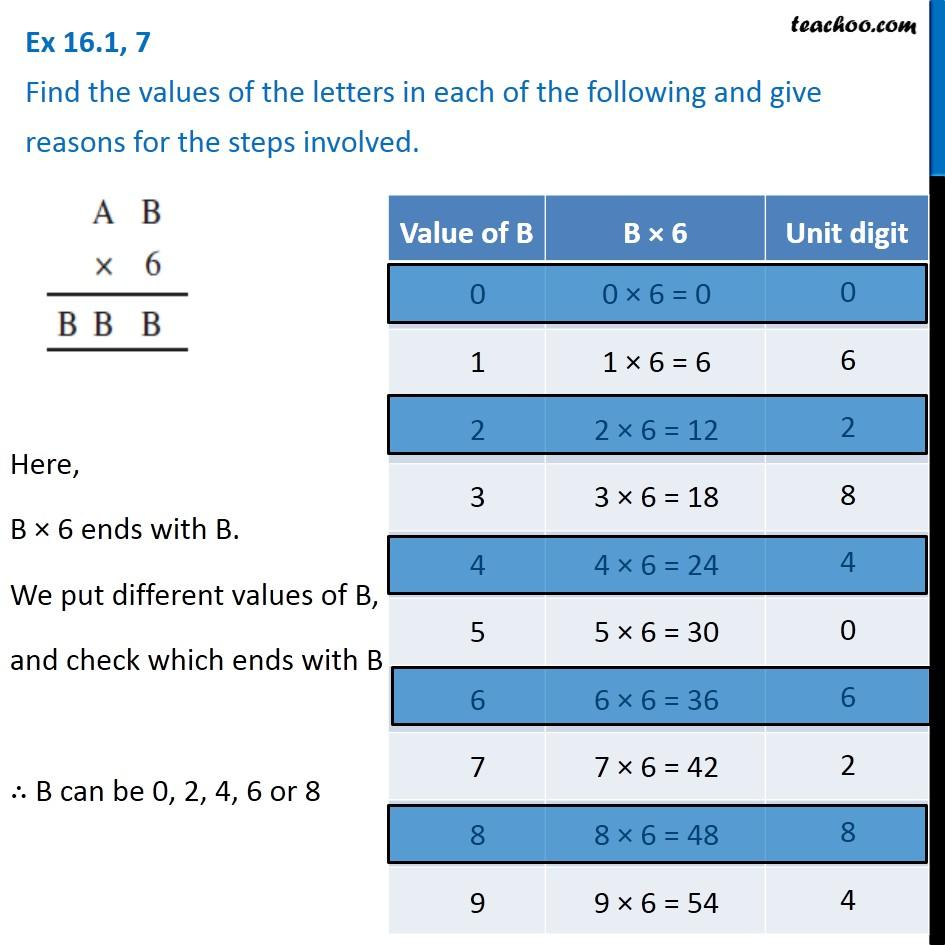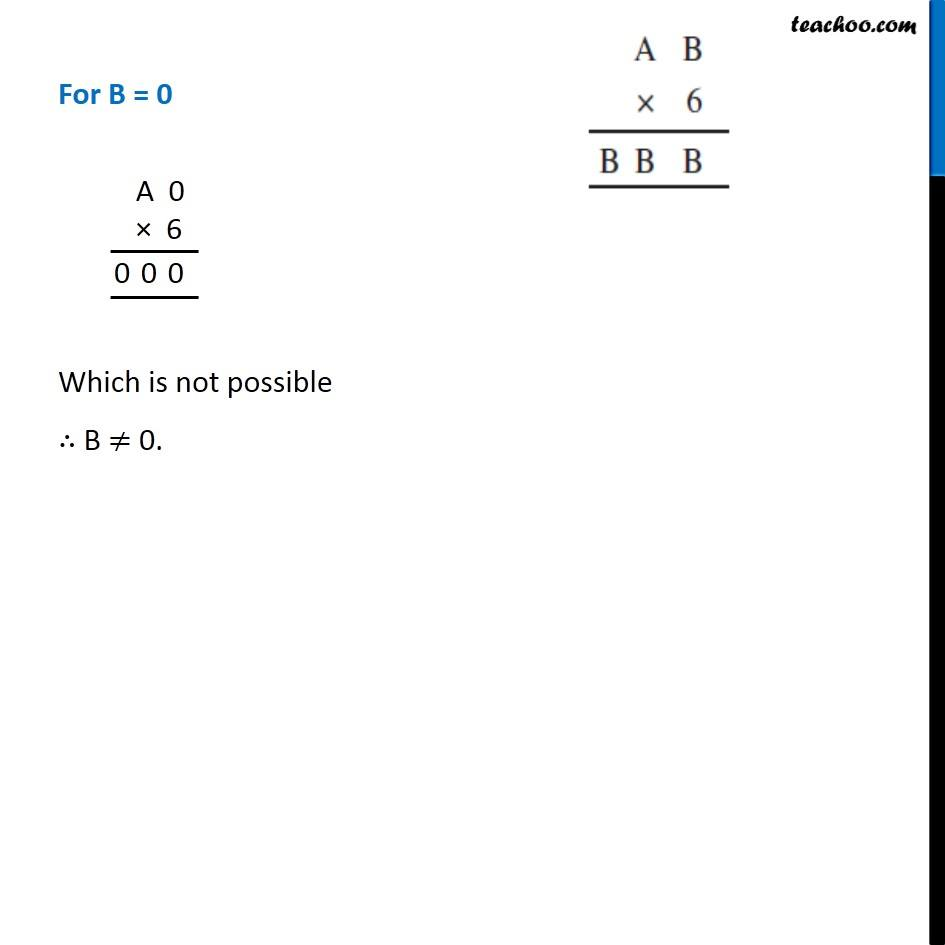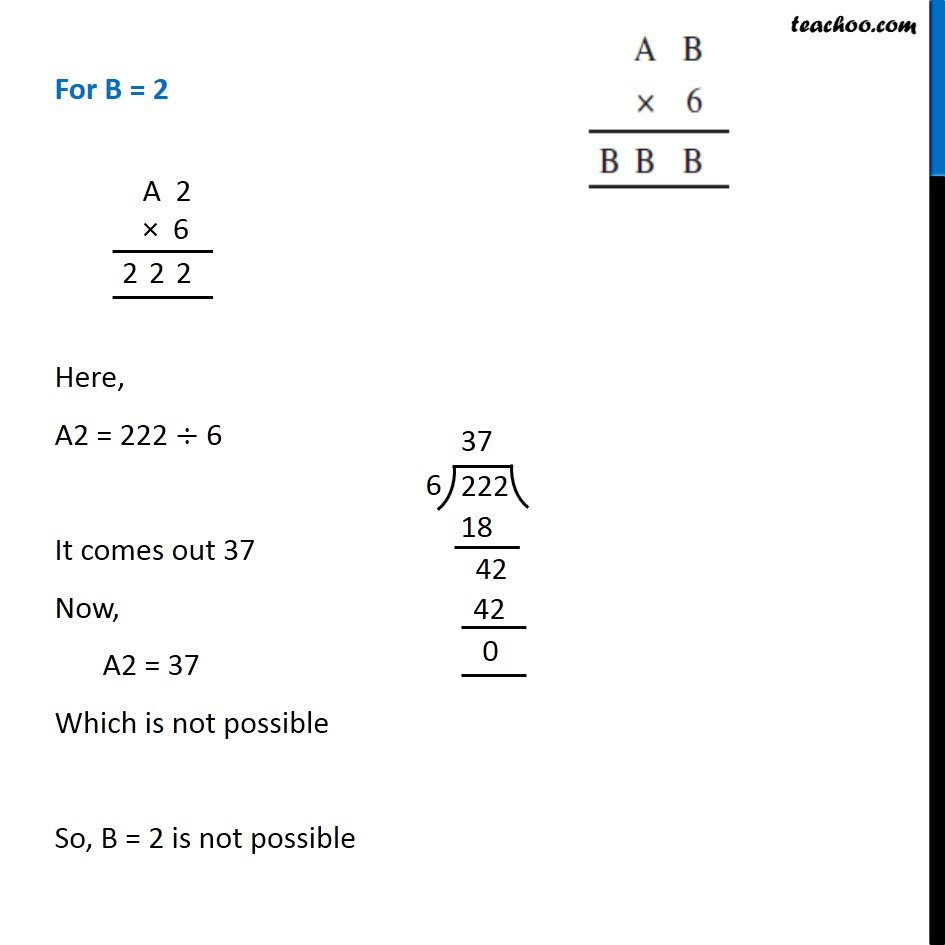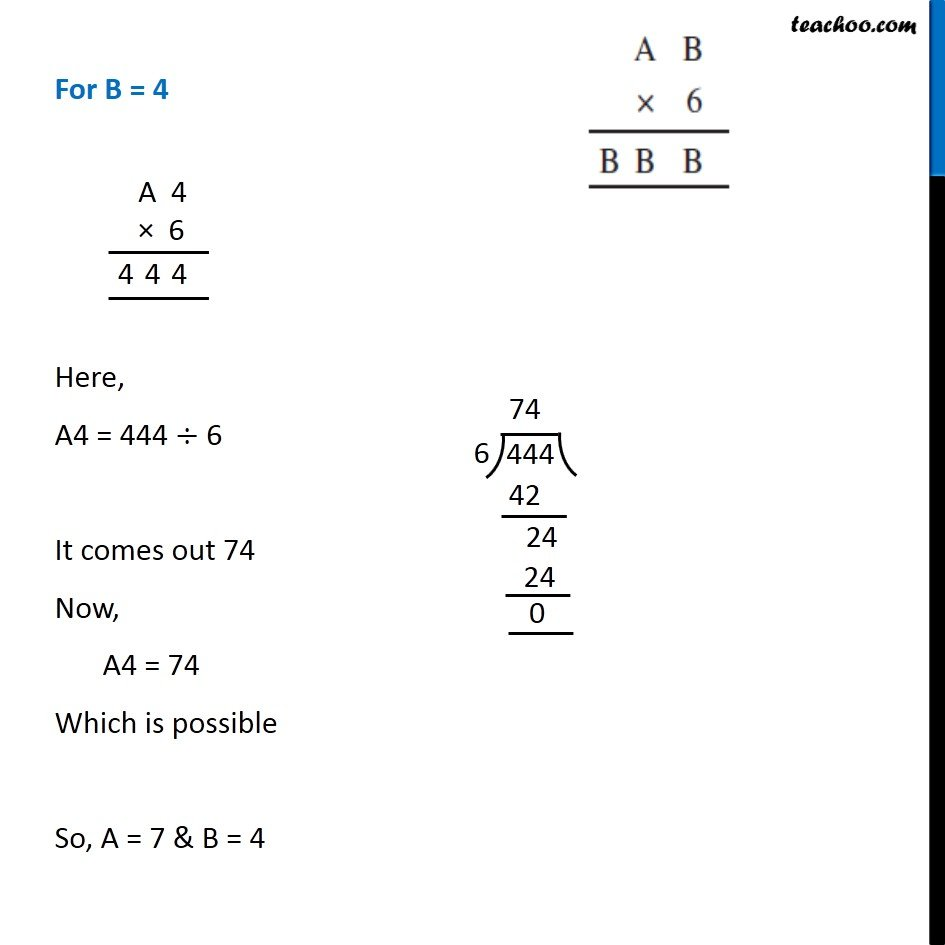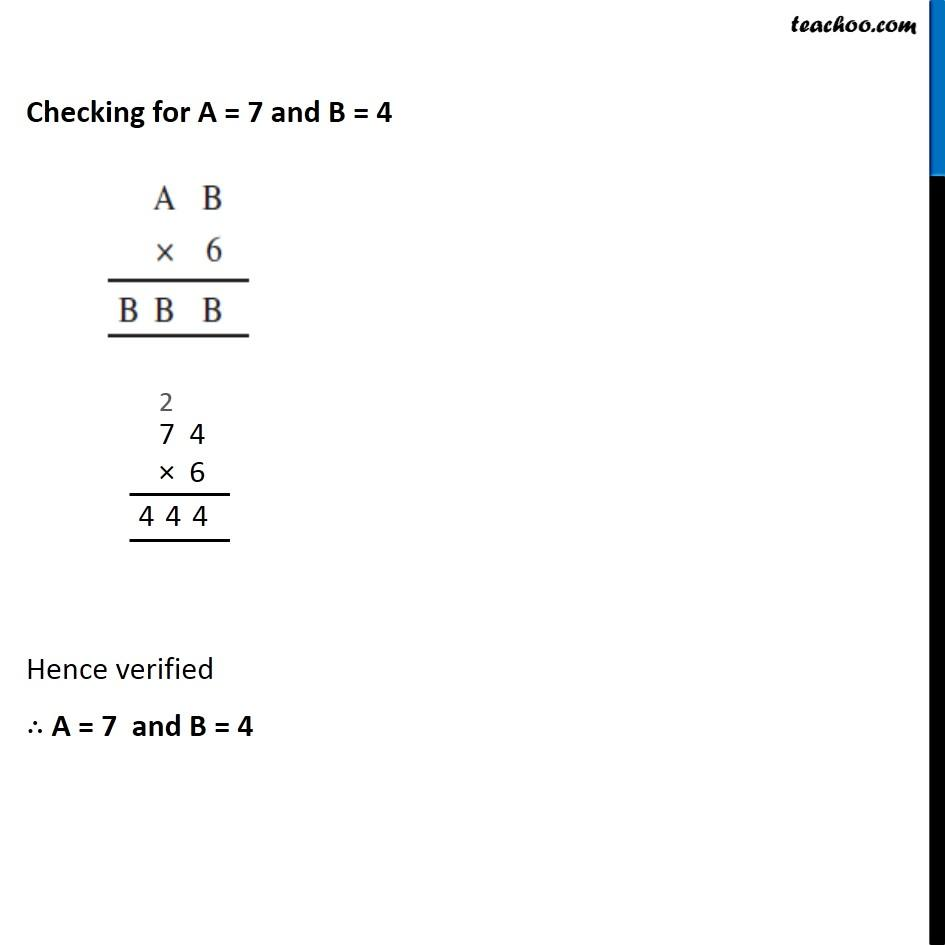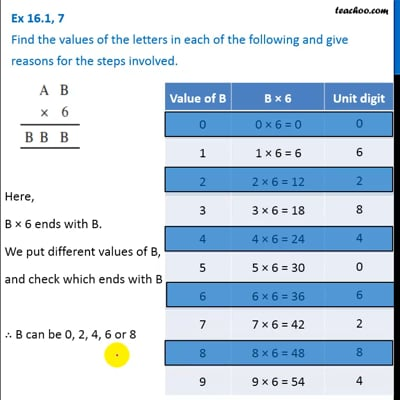This video is only available for Teachoo black users

### Transcript

Ex 16.1, 7 Find the values of the letters in each of the following and give reasons for the steps involved. Here, B × 6 ends with B. We put different values of B, and check which ends with B ∴ B can be 0, 2, 4, 6 or 8 Here, B × 6 ends with B. We put different values of B, and check which ends with B ∴ B can be 0, 2, 4, 6 or 8 For B = 0 Which is not possible ∴ B ≠ 0. For B = 2 Here, A2 = 222 ÷ 6 It comes out 37 Now, A2 = 37 Which is not possible So, B = 2 is not possible For B = 4 Here, A4 = 444 ÷ 6 It comes out 74 Now, A4 = 74 Which is possible So, A = 7 & B = 4 Checking for A = 7 and B = 4 Hence verified ∴ A = 7 and B = 4